Next: 2.3 Surface Kinetics Up: 2.2 Transport Kinetics Previous: 2.2.2 Feature-Scale Transport

## 2.2.3 Reemission

For the determination of the arrival flux distribution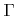the reemitted flux distribution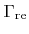must be known. This work assumes that the reemission is related to the incident flux distribution by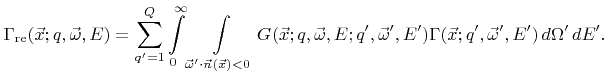(2.15)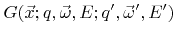is the reemission probability function which describes the conditional probability for the emission of a particle of species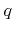and energy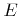into direction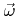, if a particle of species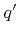, direction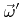, and energy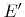strikes the surface at point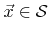.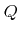is the number of process relevant particle species. This model assumes that the time between the incidence and the induced reemissions is negligible. A further assumption is that the new particles are reemitted exactly from the same location, where the incident particle has hit the surface.

The description, using the reemission probability function, is quite general in the sense that many effects can be properly treated. For example, perfect reflection of particles of typewould be described by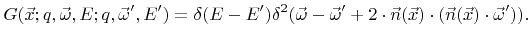(2.16)

A more realistic description of specular-like reflexions of ions is given in . If the incident direction is given by the azimuthal and the polar angle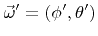relative to the surface normal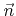withand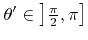, then the average reemitted direction is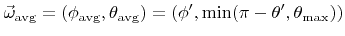. Here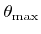is a constant parameter smaller than, but close to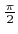. The reemitted directionis distributed around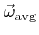according to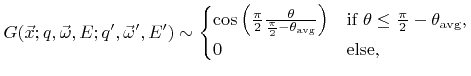(2.17)

with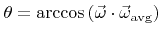.

Diffusive reemission of a neutral particle species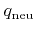is usually described by the relation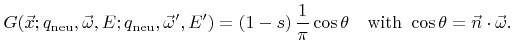(2.18)

Here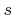is the probability that an incident particle remains sticking on the surface. The directional distribution of diffusive reemitted particles follows the Knudsen cosine law . Since the total kinetic energy of neutrals is usually given only by their thermal energy, the energy distribution of neutrals can be neglected.

The incidence of high energetic ions can lead to sputtering of particles on the surface, which are redeposited somewhere else. For example, if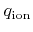denotes ions and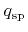denotes a sputtered particle type for which the kinetic energy can be neglected and a cosine-like directional distribution can be assumed, the reemission probability function takes the form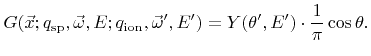(2.19)

Here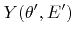is a yield function describing the average number of sputtered particles per incidence of an ion at angle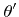and energy. Commonly, yield functions are approximated by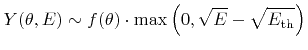(2.20)

This reflects the observed linear growth with the root of the incident energy, which must be larger than the threshold energy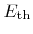to permit sputtering . The angular dependence is usually obtained by measurements. A semi-empirical formula was proposed by Yamamura et al. [76,136](2.21)

Here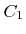and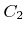are positive fitting parameters. For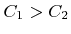this function exhibits a maximum at inclined incidence and is decreasing towards zero for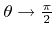.

In general, the reemission probability function may itself depend on the flux distribution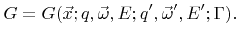(2.22)

For example, the sticking probability in (2.18) can depend on the so-called surface coverage which describes the fraction of occupied surface sites. The coverage may be influenced by the fluxes of inhibitors which adsorb on the surface and lead to some passivation of the surface. If the reemission probability function shows a dependence on the flux distributions, (2.15) will be non-linear and the calculation of the particle transport will get more complicated. This issue will be addressed in further detail in Section 5.4.1.

Finally, it should be noted that in the presence of different material regions the reemission probabilities are also dependent on the material type on the surface. As an example, the type and the number of sputtered particles vary with the material on the surface. Therefore, for each material type exposed during the process, an individual reemission probability function must be defined.Next: 2.3 Surface Kinetics Up: 2.2 Transport Kinetics Previous: 2.2.2 Feature-Scale Transport

Otmar Ertl: Numerical Methods for Topography Simulation